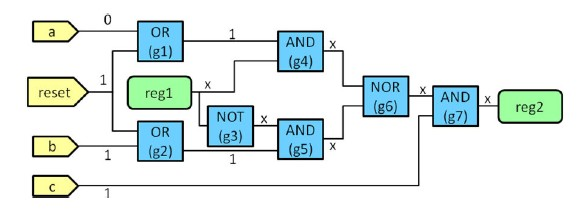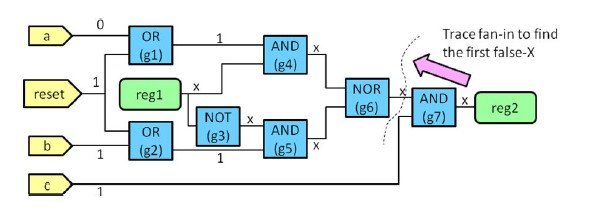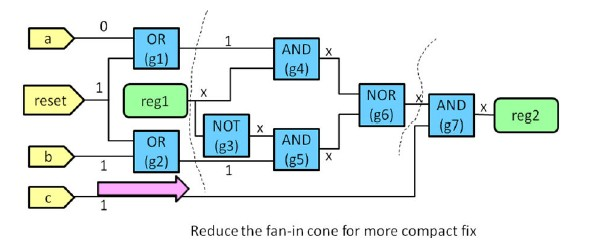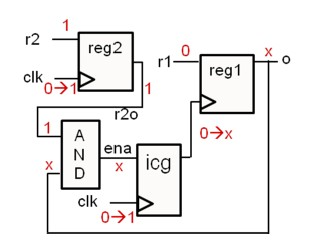# Deal with X at the Beginning and in the Middle of Simulation

Make it to the Right and Larger Audience

Blog

# Deal with X at the Beginning and in the Middle of Simulation

X can be popped in simulation in multiple ways. They may or may not be real issue.

When simulation starts, FFs without asynchronous reset will stay at X until clock comes (and if d input is non-X). But these no-reset FF outputs may cause x propagation in simulation. This may or may not be real issue. Designers need to carefully evaluate if 0 or 1 can cause issue. If not, it is good to deposit a know value, either 0 or 1, to these no-reset FFs at time 0 to get simulation going.

Another example is memory output. A memory, no matter sram or register file, normally does not have reset. So memory output is X until the corresponding memory location is written. If firmware reads a memory location before it was written, we normally treat it as real issue. Firmware engineers need to check why fw does so. However, memory output may be used to control hardware. Sometimes memory output X is not a real issue but can cause x propagation in simulation. Again, first designer needs to evaluate if 0 or 1 are both good. If so, second, we can deposit a known value at memory output at time  0 to get sim going.

Another kind of x is x pessimism. A tool such as Avery is normally adopted to deal with it. Here is some info derived from Avery doc.

Avery runs with 3rd-party simulators and provides a comprehensive set of features for addressing
issues that arise in gate-level simulation. Its most important feature is to address Xpessimism
issues in logic simulation, which is introduced in this chapter.

Different Types of Xs

We define a real X as an X in logic simulation that can have different values in real hardware, and
a false X as an X in logic simulation that can only have one value, either 0 or 1, in real hardware.
Types of Real Xs

There are two types of real Xs: functional real Xs and temporal real Xs. Functional real Xs mean
netlist values can be either 0 or 1 depending on what the initial values are when the design is
powered up. Such real Xs are usually serious and represents design issues that need to be fixed
because the circuit can be in a non-deterministic state at any given time.

Temporal real Xs mean simulation values (or netlist states) are shifted in time but otherwise
match design intentions. For example, an uninitialized clock divider can have 0!1 or 1!0 at
the first cycle. This shifts all simulation values by half cycle and the Xs are real because at a
given time, a variable can have either 0 or 1 value in real hardware. However, this may not be
a functional issue because the expected design state (simulation values) are simply shifted half
cycle. Another example is uninitialized counters that trigger some reset signals. Depending on
the power-up state of the counters, circuit reset may occur at different times. However, as long
as the reset is triggered, the design can work correctly.

SimXACT has several features to handle or trace such real Xs. See “Handling Real Xs” for
more information. For temporal real Xs, typically in RTL simulation initial blocks with random
assignments to certain FFs are added to model the power up behavior. Those initial assignments
need to be migrated to gate-level simulation as well. To this end, \$simxact rtl check with index
1 can help identify those assignments.

Types of False Xs

The most common source of false Xs in logic simulation is from X-pessimism when simulating
combinational logic. Identifying and replacing such false Xs with hardware-accurate non-X
values is the main purpose of SimXACT and will be discussed in the next section. False Xs
induced by clock gaters are also addressed by SimXACT and will be discussed in this chapter.
False Xs can also be created due to modeling issues, especially from truth tables describing
sequential element behavior such as FFs or latches. A truth table that is overly X-pessimistic
can create Xs in logic simulation that can only be 0 or 1 in real hardware. For example, a FF
may be modeled to corrupt output Q to X when clock is X and D has value change. However,
in real hardware, no matter the X on clock is 0 or 1, Q should not change when D changes
as long as the clock is stable. Therefore, the X created at the FF output is actually a false X.
SimXACT can analyze truth tables in sequential UDPs to identify such modeling issues. See

False Xs can also be sequential in nature. Sequential false Xs are Xs that have reconvergence
paths spanning multiple cycles and are resolved to a non-X value after several cycles. SimXACT
currently does not address sequential false Xs since its analysis is computationally intensive and
most design practices do not allow sequential false Xs because they are difficult to analyze and
debug.

Eliminating Combinational False Xs

The most important feature of SimXACT is to eliminate false Xs. It analyzes design logic and
the simulated trace, corrects X-pessimism in simulating combinational logic on-the-fly, and generates
behavior-level X-pessimism fixes that be used for future gate-level simulations. This technology
is patented and protected by United States Patent 8402405.

SimXACT can automatically generate behavior code for fixing gate-level X-pessimism problems.
It traces the simulated traces to find a compact sub-circuit that causes false Xs, and then
generates force/release based on the conditions to replace the false Xs with their correct constant
values that match real hardware values. This feature allows gate-level simulation to produce correct
results and is guaranteed not to mask problems because the constant values are proven by
Insights formal engine.

The following example is used to illustrate how the fix works. The initial netlist is shown belowIn the example, the “d” of “reg2” latched an X in logic simulation. However, in real circuits the
input to “reg2” is 0 because the X propagates through g4 and g3!g5 with different polarities,
making the output of g6 0.

SimXACT performs two analyses to produce compact behavior code to repair the problem. The
first analysis traces the fan-in cone of reg2.d to find the first false X:The second analysis then reduces the fan-in cone by moving the primary inputs towards reg2.d:The sub-circuit between the dotted lines is the logic responsible for generating the false X. Assume
the outputs of gates have port name “o”, then the following code will be generated to fix
gate-level simulation.
always @( g1 . o or r eg1 . q or g2 . o )
i f ( r eg1 . q === 1 ’ bx && g1 . o === 1 ’ b1 && g2 . o === 1 ’ b1 )
f o r c e g6 . o= 1 b 0 ;
e l s e
r e l e a s e g6 . o ;
The code can then be added to the original gate-level simulation to fix X-pessimism problems.

Eliminating Clock Gater False Xs

In addition to fixing X-pessimism in combinational logic, SimXACT can also fix X-pessimism
on FFs due to clock gaters. An example that shows how false Xs are generated due to clock
gaters is shown below:In this example, the X at reg1’s o is false because if the X is 0, the 0!x transition at reg1s clock
will not occur , leave reg1s value 0. If the X is 1, the transition will occur, latching the 0 at reg1’s
input. In either case, o should be updated to 0.
Unlike ordinary X-pessimism fixes, the fix has to be deposited at the registers output. Here is a
sample fix:
always @( posedge d u t . c l k )
i f ( d u t . r eg1 . q === 1 ’ bx && d u t . r1 == 1 ’ b0 && d u t . r eg2 . q == 1 ’ b1 )
begin
@( posedge d u t . c l k ) ;
\$ d e p o s i t ( d u t . r eg1 . q , 0 ) ;
endAuthor brief is empty
Groups:

Tags: